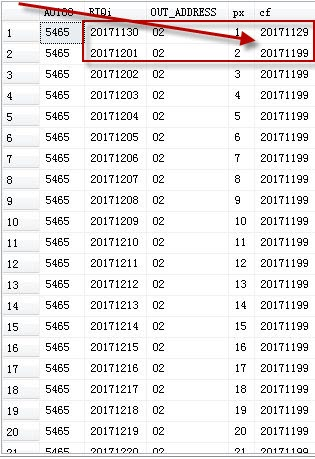MSSQL跨月连续上班统计，如何把跨月的连续天数统计在范围之内？

select A.A0188,A.RIQi,A.OUT_ADDRESS,ROW_NUMBER() OVER(PARTITION by A0188 ORDER BY riqi) as px, A.RIQi-ROW_NUMBER() OVER(PARTITION by A0188 ORDER BY riqi) as cf from
(select A0188,LEFT(CONVERT(VARCHAR(10),duty_date,112),8) as RiQi,OUT_ADDRESS from K_Day kd with (nolock) where DATEDIFF(DD,kd.duty_date,'2017-11-25')<=0 AND DATEDIFF(DD,kd.duty_date,'2017-12-24')>=0
AND (isnull(OUT_TIME1,0)+isnull(OUT_TIME2,0)+isnull(OUT_TIME3,0))>=0.1
) A...全文
228 1 点赞 打赏 收藏 举报

1 条回复

RINK_1 2017-11-17

select A.A0188,
MIN(RIQi) as start_date,
MAX(RIQi) as end_date,
cf
from
(
select A.A0188,
A.RIQi,
ROW_NUMBER() OVER(PARTITION by A0188 ORDER BY riqi) as px,
ROW_NUMBER() OVER(PARTITION by A0188 ORDER BY riqi)-ROW_NUMBER() OVER(PARTITION by A0188,OUT_ADDRESS ORDER BY riqi) as cf
from
(
select A0188,
LEFT(CONVERT(VARCHAR(10),duty_date,112),8) as RiQi,
from K_Day kd with (nolock)
where DATEDIFF(DD,kd.duty_date,'2017-11-25')<=0
AND DATEDIFF(DD,kd.duty_date,'2017-12-24')>=0
AND (isnull(OUT_TIME1,0)+isnull(OUT_TIME2,0)+isnull(OUT_TIME3,0))>=0.1
) A
) as A